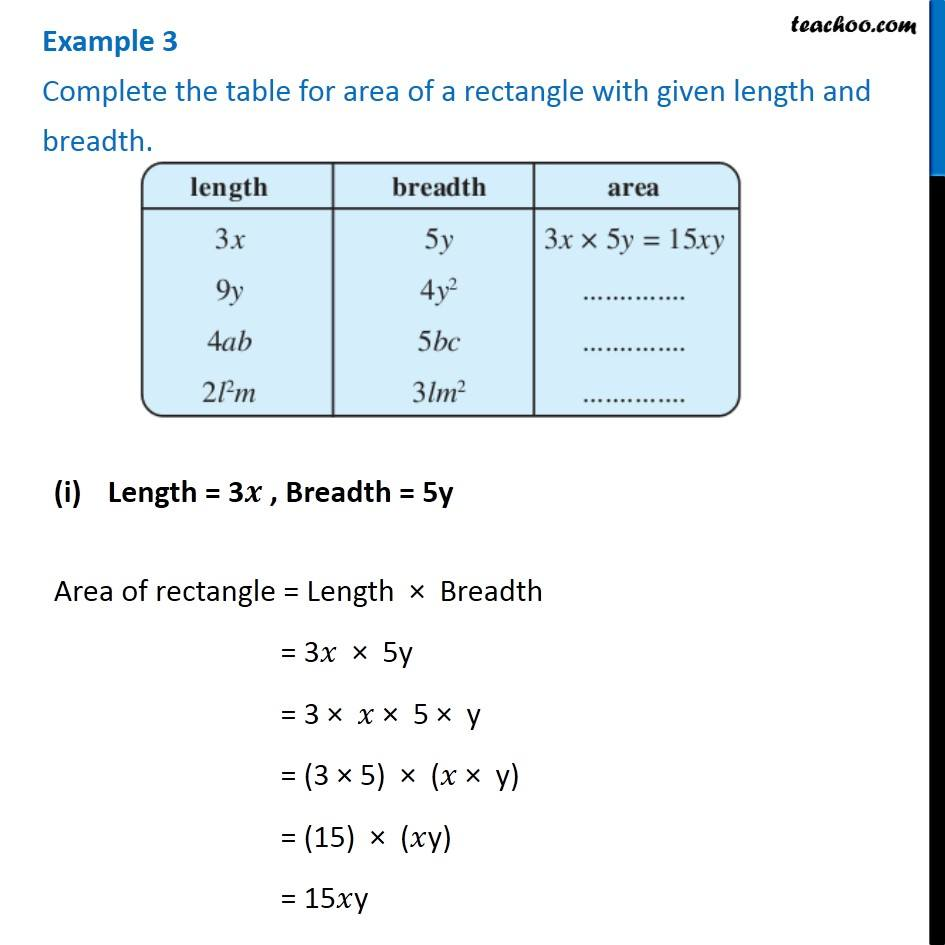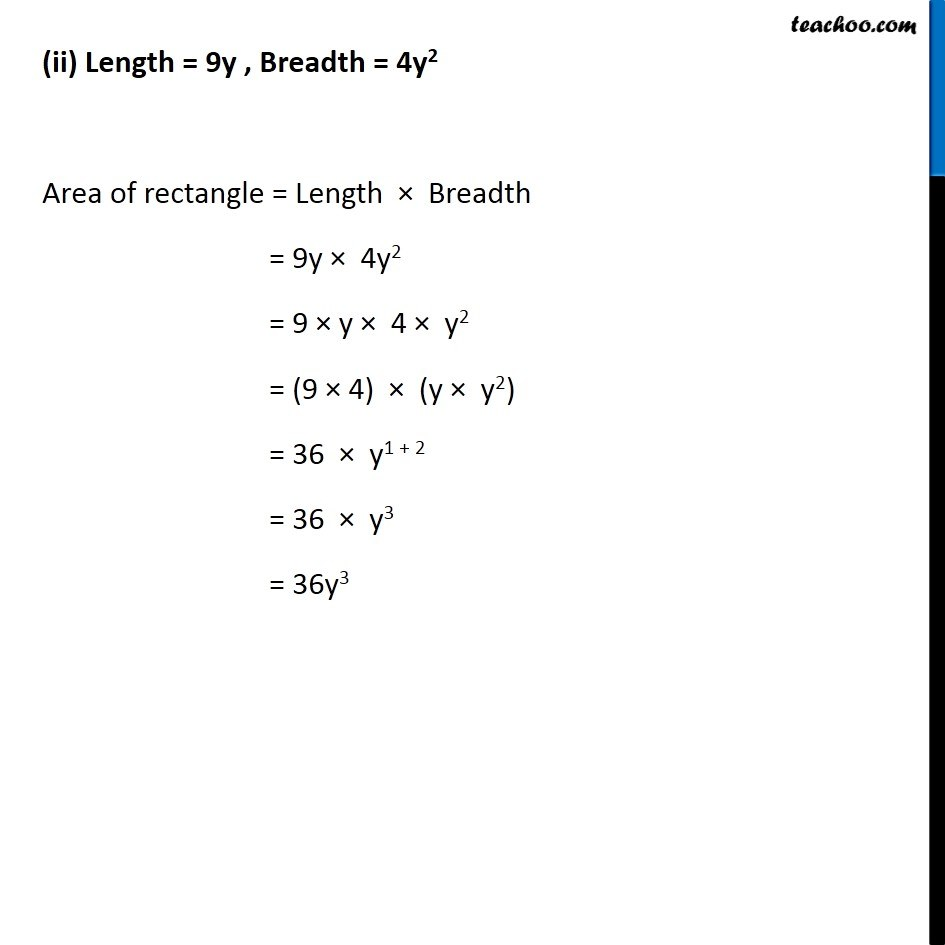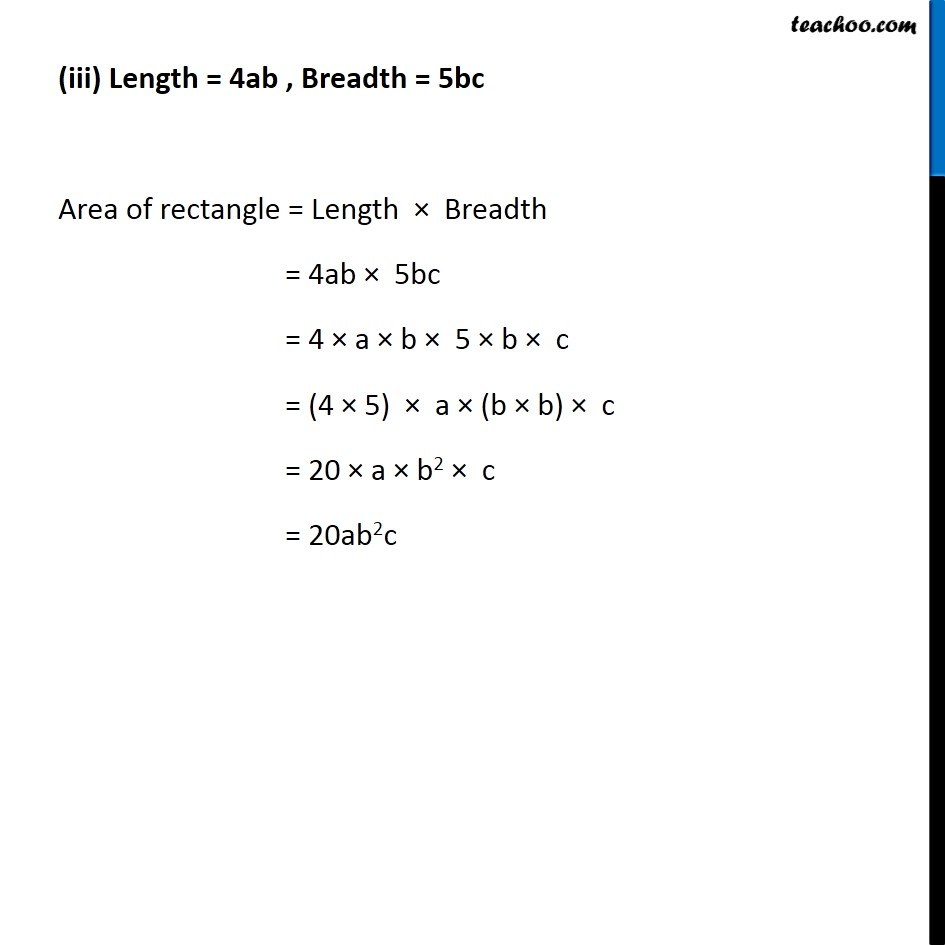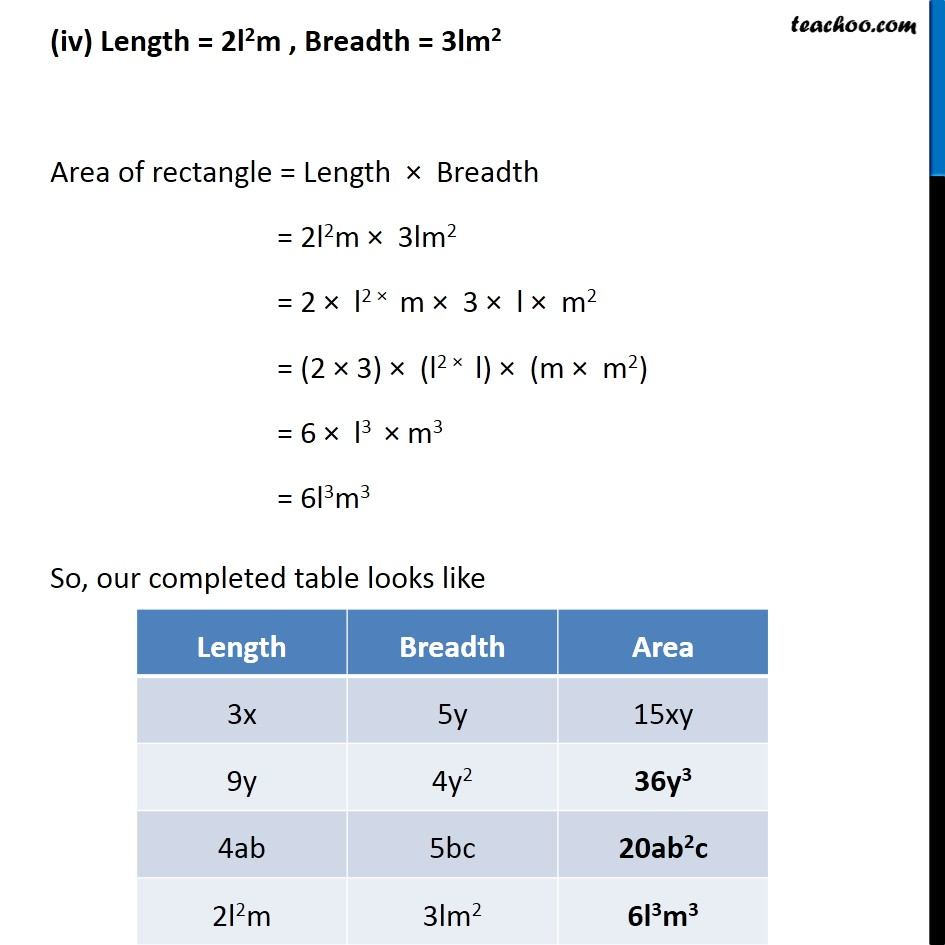1. Chapter 9 Class 8 Algebraic Expressions and Identities
2. Serial order wise
3. Examples

Transcript

Example 1 Add: 7xy + 5yz – 3zx, 4yz + 9zx – 4y , – 3xz + 5x – 2xy. Expressions are 7xy + 5yz – 3zx 4yz + 9zx – 4y – 3xz + 5x – 2xy We put like terms below like terms So, required sum = 5xy + 9yz + 3zx – 4y + 5x Example 2 Subtract 5x2 – 4y2 + 6y – 3 from 7x2 – 4xy + 8y2 + 5x – 3y. We have to subtract 1st expression from the 2nd expression. So, we write 2nd expression first. Putting like terms below like terms Example 3 Complete the table for area of a rectangle with given length and breadth. Length = 3𝒙 , Breadth = 5y Area of rectangle = Length × Breadth = 3𝑥 × 5y = 3 × 𝑥 × 5 × y = (3 × 5) × (𝑥 × y) = (15) × (𝑥y) = 15𝑥y (ii) Length = 9y , Breadth = 4y2 Area of rectangle = Length × Breadth = 9y × 4y2 = 9 × y × 4 × y2 = (9 × 4) × (y × y2) = 36 × y1 + 2 = 36 × y3 = 36y3 (ii) Length = 9y , Breadth = 4y2 Area of rectangle = Length × Breadth = 9y × 4y2 = 9 × y × 4 × y2 = (9 × 4) × (y × y2) = 36 × y1 + 2 = 36 × y3 = 36y3 (iii) Length = 4ab , Breadth = 5bc Area of rectangle = Length × Breadth = 4ab × 5bc = 4 × a × b × 5 × b × c = (4 × 5) × a × (b × b) × c = 20 × a × b2 × c = 20ab2c (iv) Length = 2l2m , Breadth = 3lm2 Area of rectangle = Length × Breadth = 2l2m × 3lm2 = 2 × l2 × m × 3 × l × m2 = (2 × 3) × (l2 × l) × (m × m2) = 6 × l3 × m3 = 6l3m3 So, our completed table looks like

Examples

About the AuthorDavneet Singh
Davneet Singh is a graduate from Indian Institute of Technology, Kanpur. He has been teaching from the past 10 years. He provides courses for Maths and Science at Teachoo.